# 【论文阅读】GAUSSIAN ERROR LINEAR UNITS (GELUS)

## Gaussian Error Linear Unit（GELU）激活函数，Sigmoid Linear Unit（SiLU）激活函数

Posted by x-jeff on April 9, 2022

# 2.GELU FORMULATION

GELU结合了dropout、zoneout以及ReLU

zoneout是用于RNN的一种正则化方法，原文：David Krueger, Tegan Maharaj, Jnos Krama ́r, Mohammad Pezeshki, Nicolas Ballas, Nan Rosemary Ke1, Anirudh Goyal, Yoshua Bengio, Hugo Larochelle, Aaron Courville, and Chris Pal. Zoneout: Regularizing RNNs by randomly preserving hidden activations. In Neural Information Processing Systems, 2016.。

GELU的定义为：

$\text{GELU} (x) = x P(X \leqslant x) = x \Phi (x) = x \frac{1}{2} \left[ 1+\text{erf} (x/ \sqrt{2}) \right]$

$\text{erf} (x)=\frac{1}{\sqrt{\pi}} \int^x_{-x} e^{-t^2} dt=\frac{2}{\sqrt{\pi}} \int^x_0 e^{-t^2} dt$

GELU可近似计算为：

$0.5x(1+ \tanh [ \sqrt{2/\pi} (x+0.044715x^3) ])$

$x\sigma (1.702x)$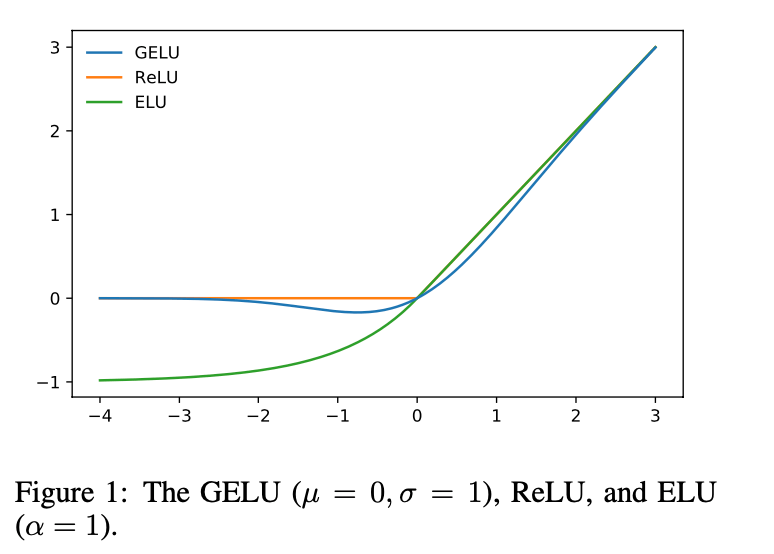# 3.GELU EXPERIMENTS

1. MNIST classification（灰度图像，10个类别，60k训练集，10k测试集）。
2. MNIST autoencoding。
3. Tweet part-of-speech tagging（训练集1000个样本，验证集327个样本，测试集500个样本）。
4. TIMIT frame recognition（训练集3696个样本，验证集1152个样本，测试集192个样本）。
5. CIFAR-10/100 classification（彩色图像，10/100个类别，50k训练集，10k测试集）。

## 3.1.MNIST CLASSIFICATION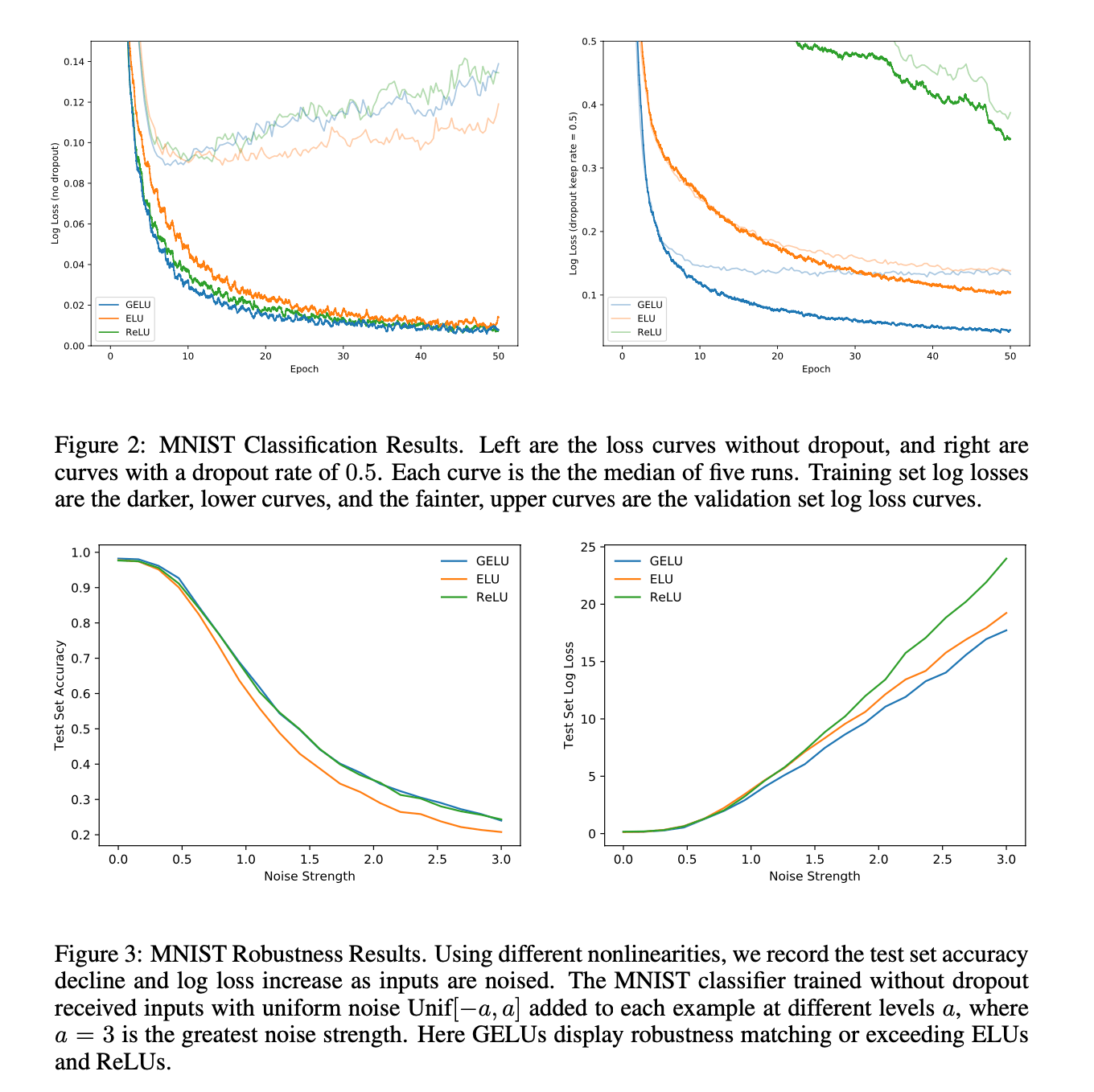## 3.2.MNIST AUTOENCODER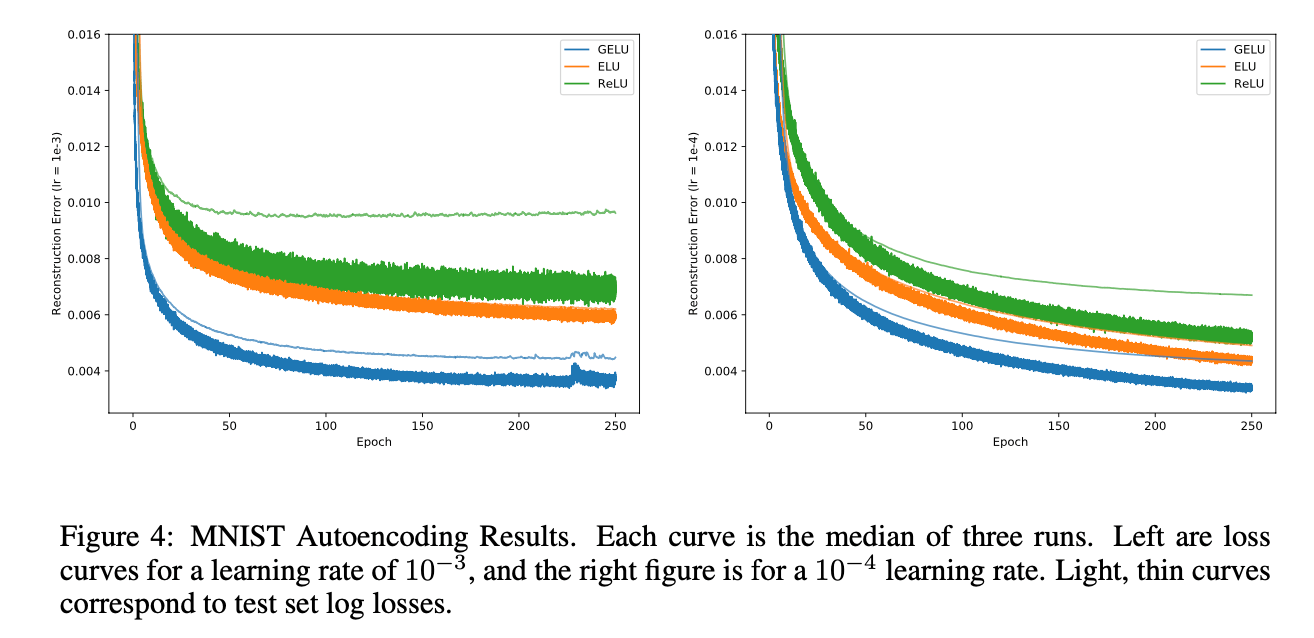NLP中的许多数据集都相对较小，所以用于NLP的激活函数也得能在小数据集上有不错的性能。在此任务上三种激活函数的test set error分别为：GELU（12.57%）、ReLU（12.67%）、ELU（12.91%）。

## 3.4.TIMIT FRAME CLASSIFICATION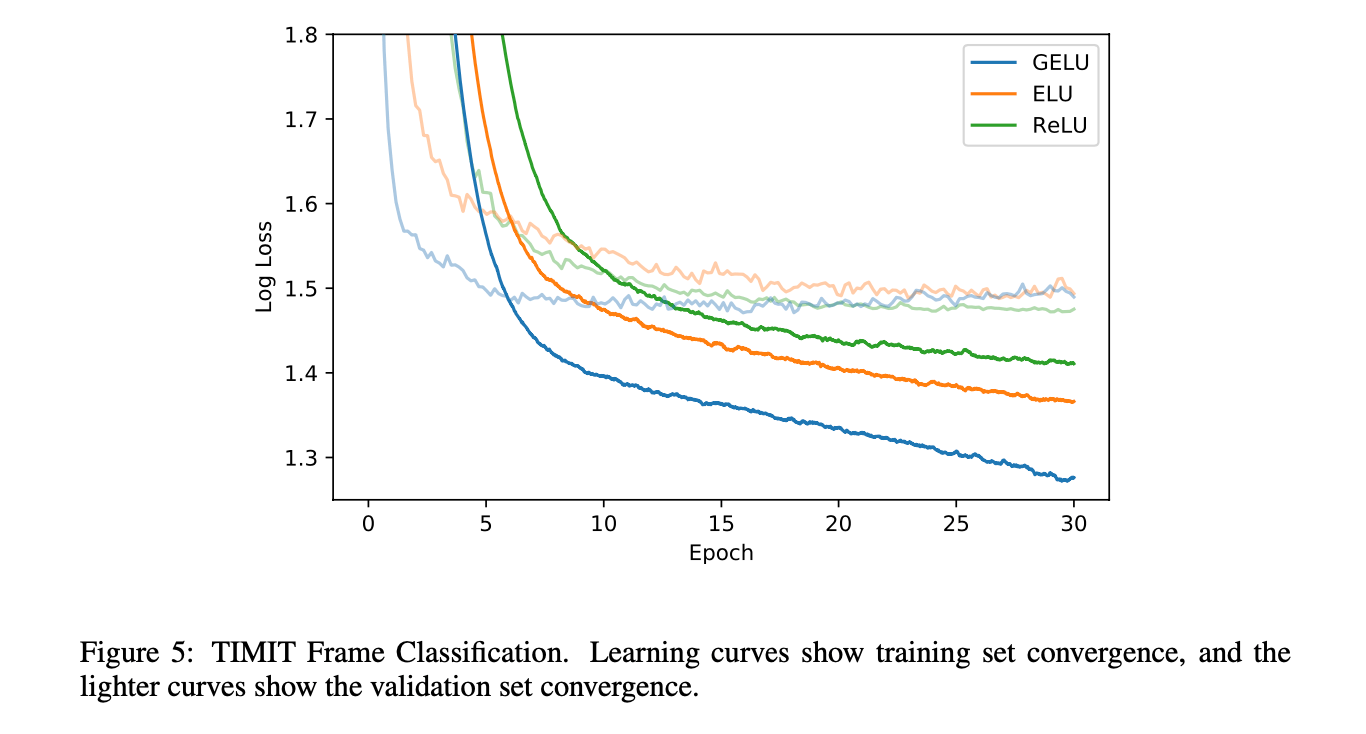## 3.5.CIFAR-10/100 CLASSIFICATION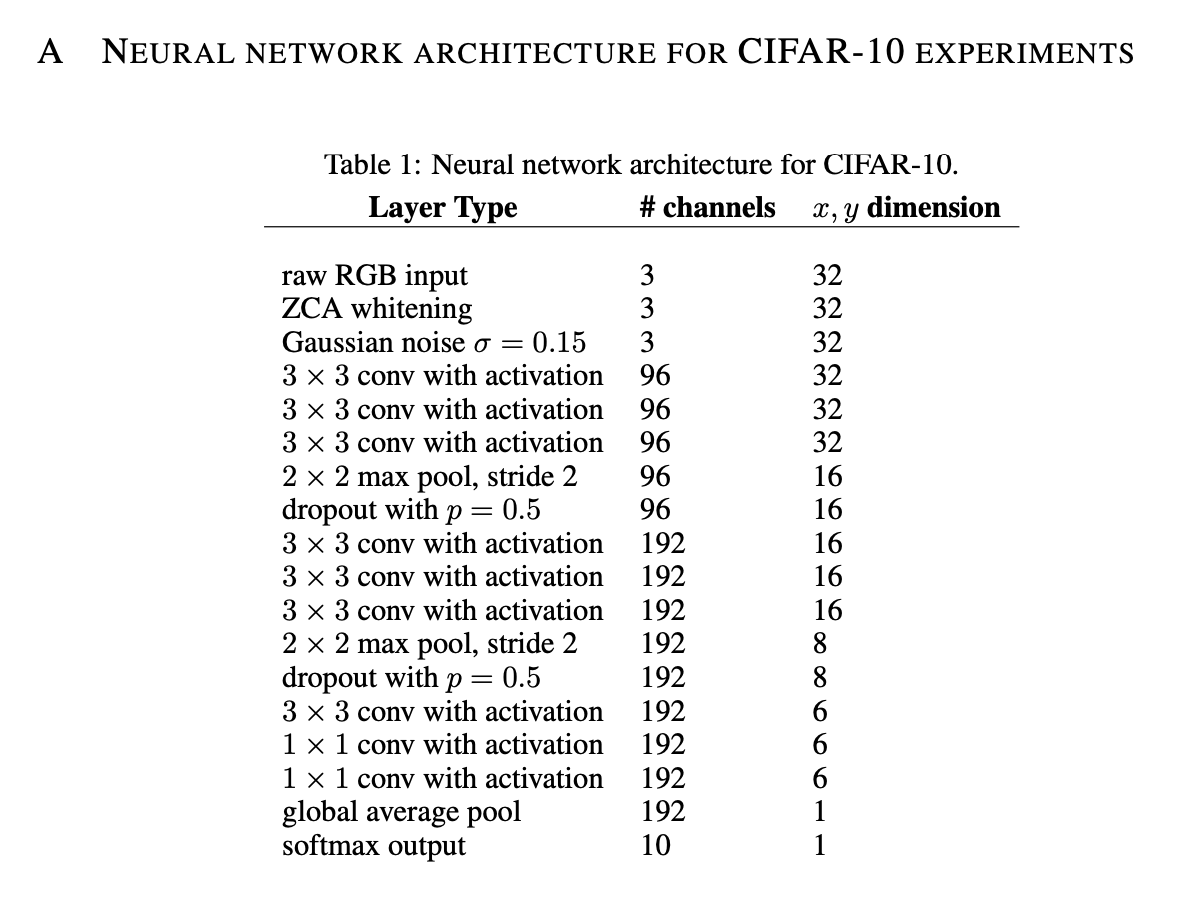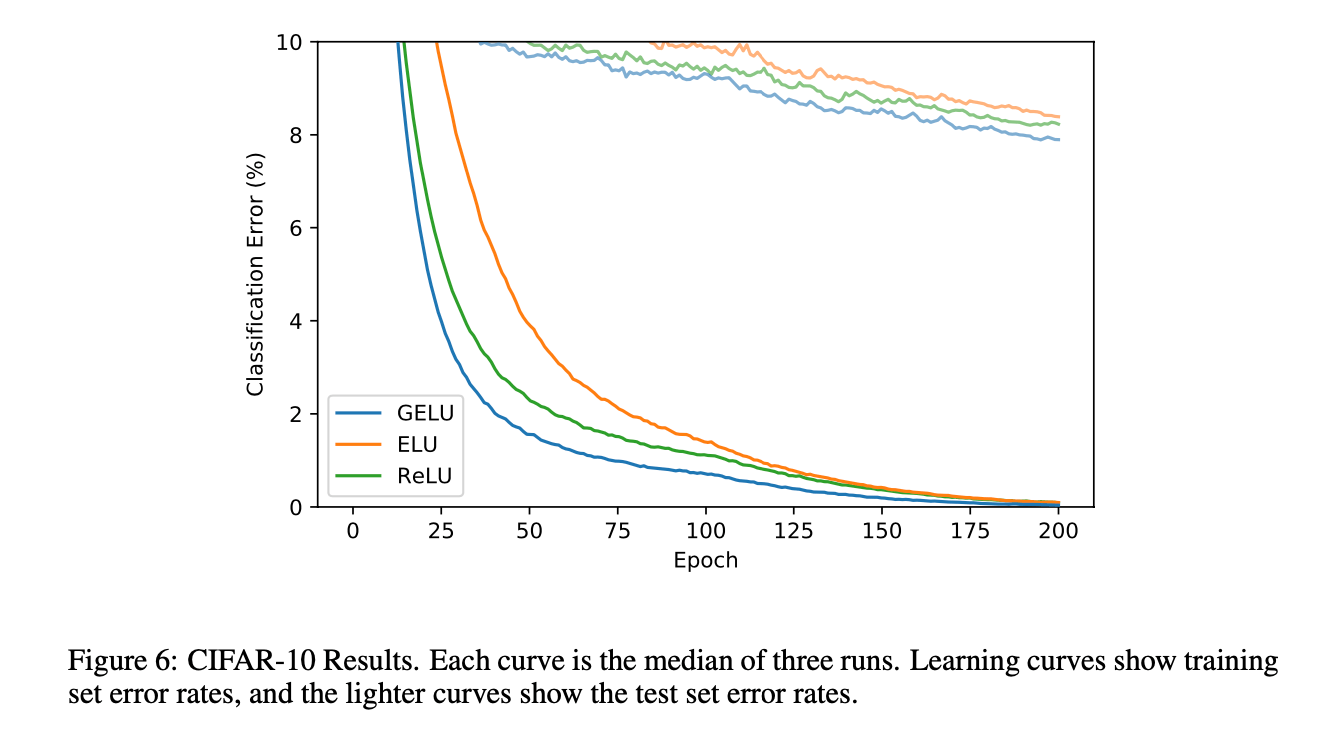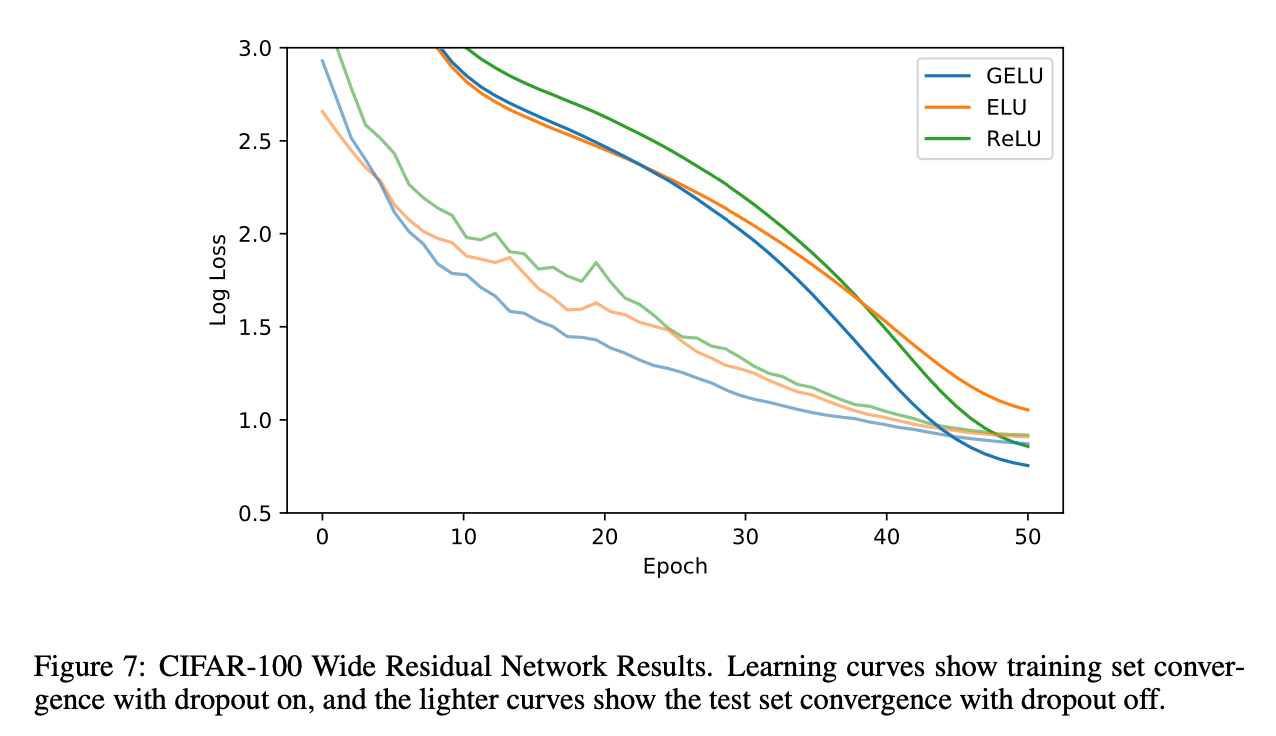# 4.DISCUSSION

GELU是非凸、非单调函数，在正值部分是非线性的。而ReLUELU是单调凸函数，在正值部分是线性的。

# 5.原文链接